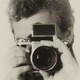## Bellows extension, exposure correction and diffraction

USING bellows or extension tubes for close-up photography moves the iris of the lens away from the camera reducing the amount of light reaching the film or the sensor.

Quite literally, the further away the light at the end of the tunnel is, the darker things are. The aperture of a tunnel entrance seen from 10 yards inside is much bigger than the aperture if you are 100 yards inside. Exactly the same applies to camera extensions.

Cameras with through-the-lens metering are measuring the amount of light actually reaching the sensor or film, so they automatically compensate for the darkening inside the tunnel.

What TTL meters don’t tell you is the actual effective aperture you are using, which affects diffraction and (less crucially) depth of field. For extreme macros using bellows or tubes it is a good idea to keep in mind the fact that the aperture the camera shows may be a whole stop or more larger than the effective aperture, so a sensor that normally starts to hit the diffraction limit at f/8 could start showing softness due to diffraction at f/5.6.

For non-TTL cameras, the main issue is calculating exposure correctly during close-up photography. The solution turns out to be simple: divide the actual distance the iris of the lens is from the camera by the focal length and you get the exposure correction factor, showing the difference between the light measured by a hand-held meter and the light reaching the film.

A 50mm lens racked out to 100mm from the film will have a correction factor of 2. At 150mm it will have a correction factor of 3. This is just the same as filter correction factors.

Filter factor 1 = no correction required

Filter factor 1.3 = 1/3 stop correction required

Filter factor 1.4  = 1/2 stop correction required

Filter factor 1.5 = 2/3 stop correction required

Filter factor 2 = 1 stop correction required

Filter factor 2.5 = 1 1/3 correction required

Filter factor 3 = 1 2/3 correction required

Filter factor 4 = 2 stops correction required

Filter factor 8 = 3 stops correction required

When calculating the effect of using extension and filters together, either add together the number of stops correction each factor requires:

Orange filter (factor 3) = 1 2/3 stop correction, + bellows extension factor 2 = 1 stop correction therefore total correction = 1 2/3 + 1 = 2 2/3

0r multiply the extension factor and the filter factor to find the overall filter factor

factor 3 x factor 2 = factor 6 = approx 2 1/2 stop correction.

The two methods don’t always give exactly the same results, presumably because the figure for the number of stops is rounded to the nearest third. The discrepancies are small but obviously would increase if three or four factors

Bellows and tubes are unlikely to produce a correction factor of more than about 3 and still have a usable working distance (e.g. 160mm of tubes on an 80mm lens), so two stops correction is likely to be the absolute maximum required. However, that would have a major impact on diffraction limits (setting the aperture at f16 to try to maximise depth of field would create an effective aperture of f32, which would cause serious diffraction blurring in a DSLR).